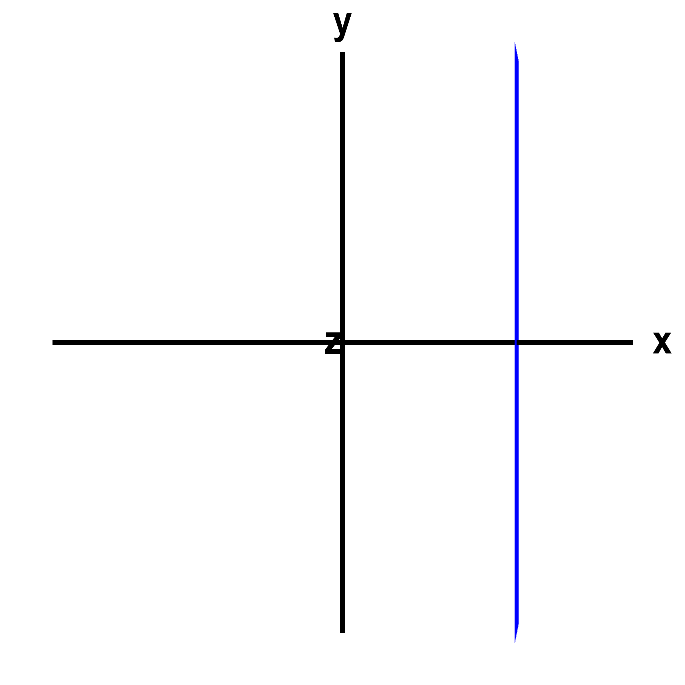# Math Insight

### Applet: A vertical line or a planeThe graph of $x=3$ would be a line, if it were an equation in two dimensions, in terms of the variables $x$ and $y$, for example. However, here it is an equation in three dimensions, in terms of the variables $x$, $y$, and $z$, so that its graph is really a plane. Rotate the graph with you mouse to see this fact. Since the equation does not depend on $y$ or $z$, the plane is parallel to both the $y$ and the $z$ axis. You can press Home to restore the original view.